# P5 Math Core Topic: Percentage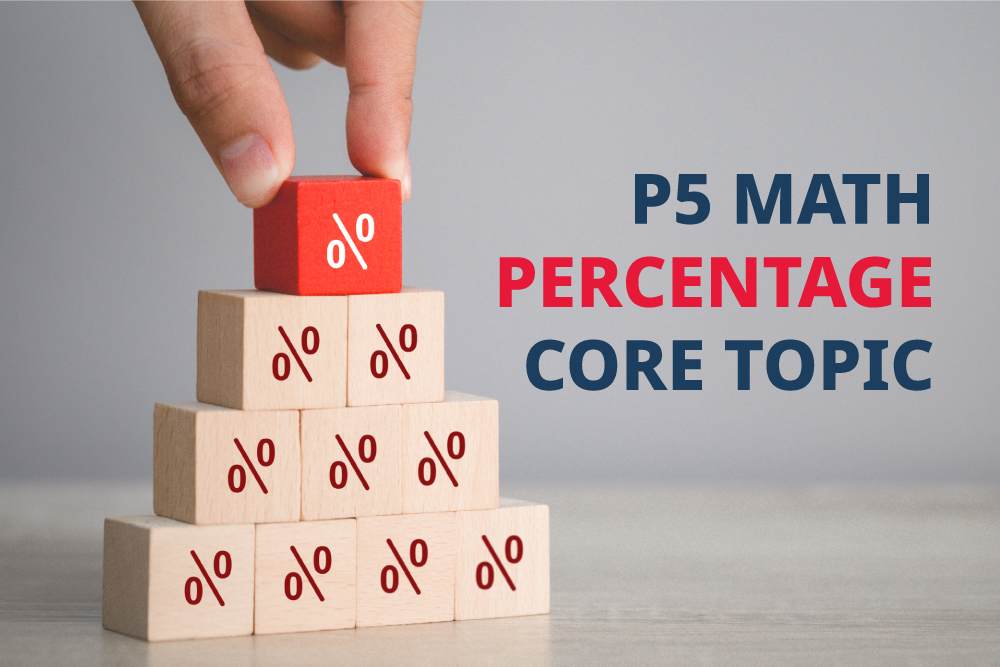## 16 Jul P5 Math Core Topic: Percentage

PERCENTAGE

Introduction

Percentage is widely used in mathematics and science topics involving data-related questions or even simple daily life situations such as discounts, profits, GST, interests, probabilities and many more. It is therefore essential for students to master the use and concepts of percentage in order to be able to fully comprehend these topics well.

Percentage is an alternative way of writing down simple fractions or ratio. It is more versatile in a sense that it provides a clearer comparison between two or more readings as shown in the following examples:

Example 1

There are 0.6 as many apples as oranges. Mrs Li bought another 1/5 of the apples. Find the percentage increase in the total number of fruits.

This question requires the student to be able to use all three concepts of fraction, ratio, and percentage together in order to solve the problem.

The first statement in the question tells the student that the number of apples is a fraction of the number of oranges. The first step is to convert 0.6 to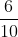, which simplifies to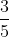. So, the number of apples isthe number of oranges. This means that there are 3 units of apples for every 5 units of oranges. This can be represented clearly in terms of ratio. It is also useful, in this case, to determine the total number of units. With 3 units of apples and 5 units of oranges, there will be a total of 8 units of fruits altogether. The next part of the questions tells us that an additional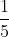of the total number of apples was bought. Hence the 3 units of apples need to be converted into a multiple of 5. We can therefore form the following ratio:

 A : O : Total = 3 : 5 : 8 (x5) 15 : 25 : 40

Apples → 15 units
Oranges → 25 units
Total → 15 + 25 = 40 units

Next, we need to determine the number of units of apples that was bought.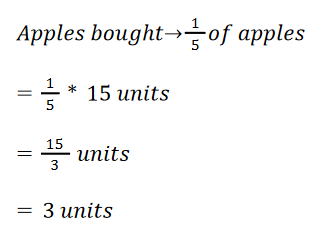The question is asking for the percentage increase in the total number of fruits. It is important to understand that the original number of fruits represents 100% and that we want to find out what is the percentage of this increase out of the original. The percentage increase can therefore be calculated by using the following formula: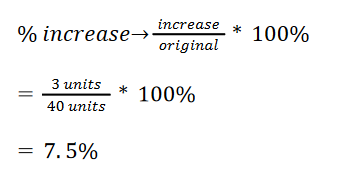This means that the number of apples that was bought is 7.5% of the total number of fruits.

Example 2

The ratio of the number of Nokia phones to the number of Samsung phones is 15 : 10.

a) What percentage of the phones are Nokia phones?

On top of the ratio given, we can add in the total number of units of phones into the ratio that we are going to work with:

 Nokia : Samsung : Total = 15 : 10 : 25

To easily form the percentage required, students need to correctly identify and extract the variables needed from the question itself. The question states: What percentage of the phones are Nokia phones? The two mentioned variables are ‘The Phones’ (The total number of phones) and ‘Nokia Phones’. ‘The Phones’ is phrased after the word ‘of’ inside the question. The value or the amount therefore goes in the denominator. The other variable ‘Nokia Phones’ will therefore go in the numerator. We therefore have: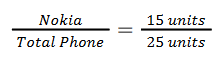To convert this fraction into percentage, the fraction needs to simply be multiplied by 100%.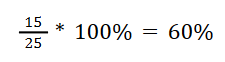This means that 60% of the all the phones are branded Nokia.

b) Given thatof the Samsung phones are black and there are thrice as many black Nokia phones as Samsung black phones, what percentage of Nokia phones are black?

Like part a, the question is asking for ‘What percentage of Nokia phones are black?’ The two variables are ‘Nokia phones’ and ‘Black (Nokia phones)’. ‘Nokia phones’ comes after the word ‘of’ and therefore will represent the denominator whereas the other variable ‘Black Nokia phones’ will be the numerator.

Before we proceed, we need to find out the number of units of black Nokia phones. We can first find out the number of units of black Samsung phones.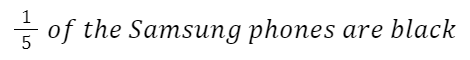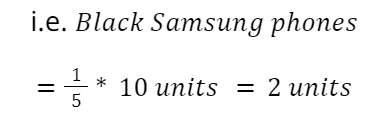There are thrice as many black Nokia phones as black Samsung phones.

i.e. Black Nokia phones = 3 * 2 units = 6 units

We then proceed to form our fraction required.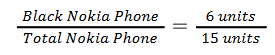Multiply the fraction by 100% to convert it to percentage.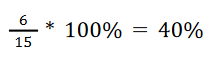This means that 40% of the Nokia phones are black.

What can Einstein do?

At Einstein, students are taught on how to break down the Math problems into simpler steps. This way, students will be able to have a more comprehensive understanding on what the question means and what is required of them to solve the question. This in turn ensures that the students will be able to follow the lessons more closely and effectively.  Students are taught to solve the Math problems using detailed methods and to sought out keywords in the questions that can help them derive the solutions correctly.  Contact Einstein now to find out how and what we can do to help your child!